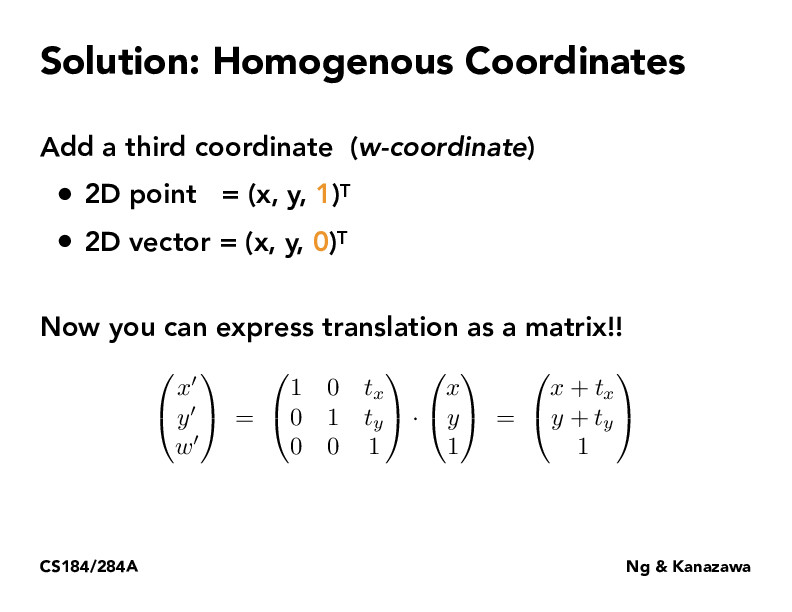Lecture 4: Transforms (23)c4554ndr4

It seems like we can only translate points and not vectors because of the zero. Is this true? Also, I was reading that w can be 2 or something here as long as x/w and y/w = x and y in the old coordinates. Is that true? Thanks!claudiakorea

I think we can translate vectors as well because the resulting matrix will be [[x + t_y], [y+t_y], ] and that would be a 2D vector as shown in the slide. During the lecture, I also believe it was mentioned that the operation here is valid as long as the w-coordinate of the result is a 1 or a 0ziyaointl

@c4554ndr4 @claudiakorea I believe translation does not have an effect on vectors because the $0$ element causess the last column of the translation matrix to be $0$ as well. It's also true that one can scale a point so that $w$ is any number. To get the original vector back, one can multiply it by $1/w$.ziyaointl

Tip: the comment section supports Latex! You can use it via double dollar signs ($[content]$).

You must be enrolled in the course to comment